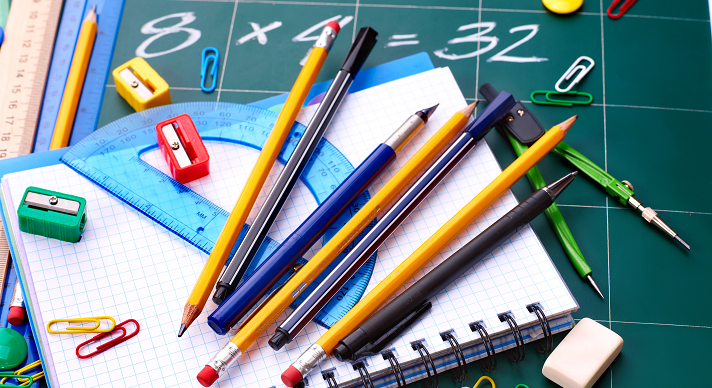### Course Overview

This Grade 4 Math course emphasizes multi-digit multiplication and division, fractions, and analysis and classification of geometric figures.

### Course Objectives & Student Learning Outcomes

Upon completion of Acellus Grade 4 Math, students will be able to: compare and order numbers; convert to roman numerals; choose a method and use the properties of addition and subtraction to add and subtract fact families and within word problems and with regrouping 3-digit and 4-digit numbers; round in the ones, thousands, and millions place; find mental sums and differences, estimations and exact answers; multiply and divide by 0 – 12 using properties of multiplication, expressions, equations, variables, order of operations, inequalities, and word problems; tell time to nearest second to include different ways to say the same time, round time, elapse time, units of time, compare units of time; and measure and find change in temperature to include reading a thermometer and negative numbers.

Students will also be able to use mental math to find products with and without regrouping; multiply 2-digit, 3-digit, and 4-digit numbers by 1 digit, and zero; multiply by tens; multiply 2-digit and 3-digit numbers with 2-digit numbers; estimate products with rounding and compatible numbers; use data and frequency tables, Venn diagrams, range, mean, median, mode, line plots, double-bar graphs, pictographs, line graphs, and circle graphs; read and make bar graphs using coordinate grid; find quotients with mental division, missing numbers, rounding, compatible numbers and larger quotients; divide 2-digit and 3-digit numbers by 1-digit numbers; divide 2-digit and 3-digit and 4-digit numbers by 2-digit numbers; choose method divide with remainders and zeroes; check division with multiplication; solve word problems; add and subtract fractions, mixed numbers, including models, number lines, equivalent fractions, simplest form; compare fractions with like denominators, with like numerators, and unlike fractions; compare mixed numbers; order fractions; change mixed numbers to improper fractions and vice versa; add and subtract like fractions, mixed numbers, and unlike denominators; and multiply whole numbers by fractions with word problems

Finally, students will be able to use number line to solve decimal problems; write decimals in expanded and word forms; convert decimals, fractions, and mixed numbers; order and round; estimate decimal sums and differences; add and subtract decimals; solve word problems; count money, convert money to decimal and fraction forms; mentally add tenths and hundredths with fractions and decimals; round to the nearest dollar; calculate change; solve story problems; identify and label points, lines, rays, angles, and polygons; classify polygons, triangles and quadrilaterals; identify circles, similar and congruent figures, symmetry, solid figures, faces, edges, and vertices; use customary as well as metric linear, weight, capacity, perimeter, area, and volume units, including changing and comparing them, and measuring fractional parts; predict outcomes; calculate probability as a fraction and mathematical and experimental probability; use tree diagrams; use divisibility rules for 2, 5, 10, and 25; find number patterns; and solve word problems.

### Scope and SequenceThis course was developed by the International Academy of Science. Learn More